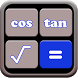# Scientific calculatorFree App

Rated 5.00/5 (3) —  Free Android application by Novel Apps and Games

★★★★★

It is time to say goodbye to your handheld scientific calculator.
Made with practicality in mind, Scientific Calculator is simple and user-friendly modern calculator app, Scientific calculator engineering app ideal for your everyday assignments. It is easy to use, large buttons, clean and stylish design, and provides the basic functionalities most people need for daily calculations. Calculator provides simple and advanced mathematical functions in a beautifully crafted with Material Design in mind. It is one of the Best simple a-Calculators which can fulfill all your calculations. If you have an Android Wear, use this Scientific calculator Casio original anytime. Scientific calculator Casio fx 100 mx Simple calculator.

Scientific Calculator has the "expression" mode where you can write expressions in a natural way and watch your calculations. When possible, an expression in this advanced calculator is simplified and the result is displayed as an expression using fractions, square roots and constant. Enjoy calculating calculatrice devise for free with various solver features as exponent calculator fraction Calculator or a Cut Calculator.
Daily Base Usage :

For example, Calculator is perfect in situations such as adding up receipts, calculating taxes or discounts when shopping, doing homework study for school, some calculation at your workplace, or even when you calculate a tip at restaurants.
This calculator Comprises of all modern and advanced features of latest generations Calculators.

◆ Supports basic calculations such as addition, subtraction, multiplication, and division.
◆ Supports advanced calculations with scientific operations.
◆ Supports editing a formula during the input.
◆ Real equation view editor with brackets and operator priority support.
◆ Component or polar complex entry/view mode.
◆ Physical, mathematical, chemical calculations.
◆ Fixed, scientific and engineering view mode.
◆ Perform mathematical operations for mathematical equations.
◆ For engineering or graphical calculations.
◆ Basic arithmetic operations including percentage, modulo and negation.
◆ Periodic numbers and their conversion to fractions.
◆ Repeated operations.
◆ Complex numbers.
◆ Conversion between rectangular and polar coordinates.
◆ Advanced number operations such as random numbers, combinations, permutations, common greatest divisor, etc.
◆ Trigonometric and hyperbolic functions.
◆ Powers, roots, logarithms, etc.
◆ Fixed point, scientific and engineering display format.
◆ Display exponent as SI units prefix.
◆ Binary, octal and hexadecimal numeral systems.
◆ Logical operations.
◆ Bitwise shifts and rotations.
◆ Haptic feedback.

If you want to send us your comments or suggestions about our products, we really will listen to what you have to say, you can help us decide what are the most important things to work on with the next version? Help us to help you, and you could see your suggestions included in the next release!

Be proud using our app!

Free
downl.
Android
3.0+
For everyone
Android app

R70%
by R####:

best app

RATING
5.05
3 users

5

4

3

2

1